# Search by Topic

#### Resources tagged with Simultaneous equations similar to System Speak:

Filter by: Content type:
Age range:
Challenge level:

### There are 40 results

Broad Topics > Algebraic expressions, equations and formulae > Simultaneous equations### System Speak

##### Age 16 to 18 Challenge Level:

Five equations... five unknowns... can you solve the system?### Matchless

##### Age 14 to 16 Challenge Level:

There is a particular value of x, and a value of y to go with it, which make all five expressions equal in value, can you find that x, y pair ?### Always Two

##### Age 14 to 18 Challenge Level:

Find all the triples of numbers a, b, c such that each one of them plus the product of the other two is always 2.### Leonardo's Problem

##### Age 14 to 18 Challenge Level:

A, B & C own a half, a third and a sixth of a coin collection. Each grab some coins, return some, then share equally what they had put back, finishing with their own share. How rich are they?### Pair Squares

##### Age 16 to 18 Challenge Level:

The sum of any two of the numbers 2, 34 and 47 is a perfect square. Choose three square numbers and find sets of three integers with this property. Generalise to four integers.##### Age 16 to 18 Challenge Level:

Find all positive integers a and b for which the two equations: x^2-ax+b = 0 and x^2-bx+a = 0 both have positive integer solutions.### Which Is Cheaper?

##### Age 14 to 16 Challenge Level:

When I park my car in Mathstown, there are two car parks to choose from. Can you help me to decide which one to use?##### Age 14 to 16 Challenge Level:

Four jewellers share their stock. Can you work out the relative values of their gems?### All-variables Sudoku

##### Age 11 to 18 Challenge Level:

The challenge is to find the values of the variables if you are to solve this Sudoku.### Pareq Calc

##### Age 14 to 16 Challenge Level:

Triangle ABC is an equilateral triangle with three parallel lines going through the vertices. Calculate the length of the sides of the triangle if the perpendicular distances between the parallel. . . .### Real(ly) Numbers

##### Age 16 to 18 Challenge Level:

If x, y and z are real numbers such that: x + y + z = 5 and xy + yz + zx = 3. What is the largest value that any of the numbers can have?### Rudolff's Problem

##### Age 14 to 16 Challenge Level:

A group of 20 people pay a total of £20 to see an exhibition. The admission price is £3 for men, £2 for women and 50p for children. How many men, women and children are there in the group?### Multiplication Arithmagons

##### Age 14 to 16 Challenge Level:

Can you find the values at the vertices when you know the values on the edges of these multiplication arithmagons?### LCM Sudoku II

##### Age 11 to 18 Challenge Level:

You are given the Lowest Common Multiples of sets of digits. Find the digits and then solve the Sudoku.### Arithmagons

##### Age 14 to 16 Challenge Level:

Can you find the values at the vertices when you know the values on the edges?### Overturning Fracsum

##### Age 14 to 16 Challenge Level:

Solve the system of equations to find the values of x, y and z: xy/(x+y)=1/2, yz/(y+z)=1/3, zx/(z+x)=1/7### Escriptions

##### Age 16 to 18 Challenge Level:

For any right-angled triangle find the radii of the three escribed circles touching the sides of the triangle externally.### Coffee

##### Age 14 to 16 Challenge Level:

To make 11 kilograms of this blend of coffee costs £15 per kilogram. The blend uses more Brazilian, Kenyan and Mocha coffee... How many kilograms of each type of coffee are used?### Negatively Triangular

##### Age 14 to 16 Challenge Level:

How many intersections do you expect from four straight lines ? Which three lines enclose a triangle with negative co-ordinates for every point ?### Polycircles

##### Age 14 to 16 Challenge Level:

Show that for any triangle it is always possible to construct 3 touching circles with centres at the vertices. Is it possible to construct touching circles centred at the vertices of any polygon?### CD Heaven

##### Age 14 to 16 Challenge Level:

All CD Heaven stores were given the same number of a popular CD to sell for £24. In their two week sale each store reduces the price of the CD by 25% ... How many CDs did the store sell at. . . .### Intersections

##### Age 14 to 18 Challenge Level:

Change one equation in this pair of simultaneous equations very slightly and there is a big change in the solution. Why?### Fruity Totals

##### Age 7 to 16 Challenge Level:

In this interactivity each fruit has a hidden value. Can you deduce what each one is worth?### Walls

##### Age 16 to 18 Challenge Level:

Plane 1 contains points A, B and C and plane 2 contains points A and B. Find all the points on plane 2 such that the two planes are perpendicular.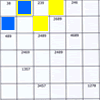### Intersection Sudoku 2

##### Age 11 to 16 Challenge Level:

A Sudoku with a twist.### Warmsnug Double Glazing

##### Age 14 to 16 Challenge Level:

How have "Warmsnug" arrived at the prices shown on their windows? Which window has been given an incorrect price?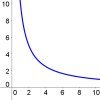### Graphs of Changing Areas

##### Age 16 to 18 Challenge Level:

Use graphs to gain insights into an area and perimeter problem, or use your knowledge of area and perimeter to gain insights into the graphs...### Building Tetrahedra

##### Age 14 to 16 Challenge Level:

Can you make a tetrahedron whose faces all have the same perimeter?### How Many Balls?

##### Age 16 to 18 Challenge Level:

A bag contains red and blue balls. You are told the probabilities of drawing certain combinations of balls. Find how many red and how many blue balls there are in the bag.### Which Is Bigger?

##### Age 14 to 16 Challenge Level:

Which is bigger, n+10 or 2n+3? Can you find a good method of answering similar questions?### Surds

##### Age 14 to 16 Challenge Level:

Find the exact values of x, y and a satisfying the following system of equations: 1/(a+1) = a - 1 x + y = 2a x = ay##### Age 11 to 16 Challenge Level:

Four numbers on an intersection that need to be placed in the surrounding cells. That is all you need to know to solve this sudoku.### Double Time

##### Age 16 to 18 Challenge Level:

Crack this code which depends on taking pairs of letters and using two simultaneous relations and modulus arithmetic to encode the message.### What's it Worth?

##### Age 11 to 16 Challenge Level:

There are lots of different methods to find out what the shapes are worth - how many can you find?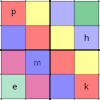### Simultaneous Equations Sudoku

##### Age 11 to 16 Challenge Level:

Solve the equations to identify the clue numbers in this Sudoku problem.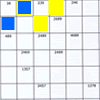### Intersection Sudoku 1

##### Age 11 to 16 Challenge Level:

A Sudoku with a twist.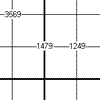##### Age 11 to 16 Challenge Level:

This is a variation of sudoku which contains a set of special clue-numbers. Each set of 4 small digits stands for the numbers in the four cells of the grid adjacent to this set.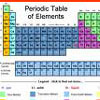### A Method of Defining Coefficients in the Equations of Chemical Reactions

##### Age 14 to 18

A simple method of defining the coefficients in the equations of chemical reactions with the help of a system of linear algebraic equations.### Cobalt Decay

##### Age 16 to 18 Challenge Level:

Investigate the effects of the half-lifes of the isotopes of cobalt on the mass of a mystery lump of the element.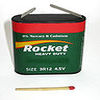### Battery Modelling

##### Age 16 to 18 Challenge Level:

Find out how to model a battery mathematically